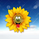477浏览
//@version=3

m = ema (close, 34)
pm = ema (close, 34)
t = ema (close, 144)
pt = ema (close, 144)
prev = pm - pt
curr = m - t

plot(m, "Momentum", blue, transp=0)
plot(t, "Trend", red, transp=0)

cross_up = prev <= 0 and curr > 0
cross_down = prev >= 0 and curr < 0

## 评论